# OpenGL Diary

## Day 2 - Configuring the projection

The next thing is to figure out how the Z coordinate affects the view drawn by OpenGL. So lets modifiy the draw function.

void draw() {

glClear(GL_COLOR_BUFFER_BIT | GL_DEPTH_BUFFER_BIT);

glBegin(GL_TRIANGLES);

for (float i = 0.0f; i < 1.5f; i += 0.3f) {

glVertex3f(-0.6f + i, -0.6f + i, -1.2f + i * 2);

glVertex3f(-0.4f + i, -0.6f + i, -1.2f + i * 2);

glVertex3f(-0.4f + i, -0.4f + i, -1.2f + i * 2);

}

glEnd();

glFlush();

glutSwapBuffers();

}

Running this new version will give the following picture.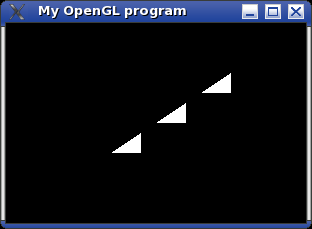There are two things which become apparent. The first (z = -1.2) and last (z = 1.2) triangle are not drawn and all drawn triangles are the same size.

This is because OpenGL by default projects a cube with side length of 2 centered at the origin onto the viewport. The projection used is also an orthographic one, which causes objects at any distance to be drawn the same size.

To change this, one has to modify the projection matrix like in the following example.

void draw() {

glMatrixMode(GL_PROJECTION);

gluPerspective(55.0, 1.0, 1.0, 10.0);

glMatrixMode(GL_MODELVIEW);

glClear(GL_COLOR_BUFFER_BIT | GL_DEPTH_BUFFER_BIT);

glBegin(GL_TRIANGLES);

for (float i = 0.0f; i < 1.5f; i += 0.3f) {

glVertex3f(-0.6f + i, -0.6f + i, -1.3f - i * 2);

glVertex3f(-0.4f + i, -0.6f + i, -1.3f - i * 2);

glVertex3f(-0.4f + i, -0.4f + i, -1.3f - i * 2);

}

glEnd();

glFlush();

glutSwapBuffers();

}

Resulting in the following output.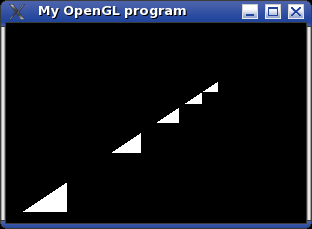Yet again a function was used (gluPerspective) that is obviously not really part of OpenGL, but belongs to GLU. This is just an easier way to set the projection matrix than to use glFrustum.

Common trap:

When modifying the projection the matrix mode must be set to GL_PROJECTION using the glMatrixMode function. However most of the time the matrix mode GL_MODELVIEW is used.

So I found it useful to always set the matrix mode back to GL_MODELVIEW as soon as possible. This way you can always be sure this mode is active.

Whe can also notice that a negative Z coordinate places the object in front of the "camera" and decreasing the Z coordinate causes the object to appear further away. The eyepoint is placed at the origin, so positive Z coordinates are "behind the camera".

Now if you resize the window, something odd will happen.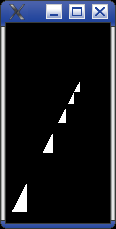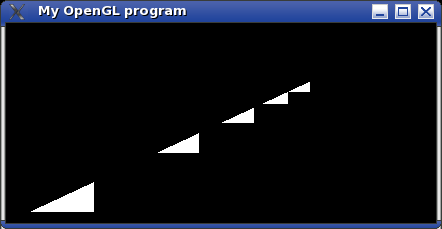This is because gluPerspective fits the given field of view (first parameter, 55 degrees in my example) into the window regardless of size. To change this one has to specify the ratio (second parameter, always 1.0 in my example) of the window width towards the window height.

However this has to be done everytime the window is resized, so GLUT provides with a way to specify a function that is called when this happens, by passing the name of the function to glutReshapeFunc. Now the basic program looks like this.

void reshape(int width, int height) {

glViewport(0, 0, width, height);

float ratio = ((float) width) / height;

glMatrixMode(GL_PROJECTION);

gluPerspective(55.0, ratio, 1.0, 10.0);

glMatrixMode(GL_MODELVIEW);

}

void draw() {

glClear(GL_COLOR_BUFFER_BIT | GL_DEPTH_BUFFER_BIT);

glBegin(GL_TRIANGLES);

for (float i = 0.0f; i < 1.5f; i += 0.3f) {

glVertex3f(-0.6f + i, -0.6f + i, -1.3f - i * 2);

glVertex3f(-0.4f + i, -0.6f + i, -1.3f - i * 2);

glVertex3f(-0.4f + i, -0.4f + i, -1.3f - i * 2);

}

glEnd();

glFlush();

glutSwapBuffers();

}

int main(int argc, char* argv[]) {

glutInit(&argc, argv);

glutInitDisplayMode(GLUT_RGBA | GLUT_DEPTH | GLUT_DOUBLE);

glutInitWindowPosition(100, 100);

glutInitWindowSize(300, 200);

glutCreateWindow("My OpenGL program");

glutDisplayFunc(draw);

glutIdleFunc(draw);

glutReshapeFunc(reshape);

glutMainLoop();

}

The call to glViewport is necessary so OpenGL uses the complete window for drawing.

The result we get is that the window height now spans the given field of view, while the window width shows an apropriate field of view. Now the triangles are no longer distorted with the two shorter sides beeing the same length.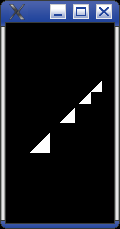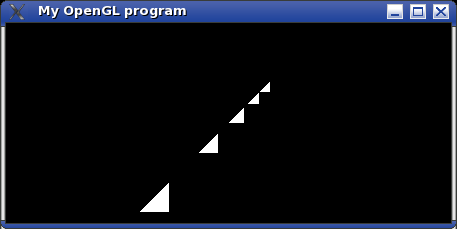On Day 3 - Placing things into the world we will see how to put objects into the "virtual world".